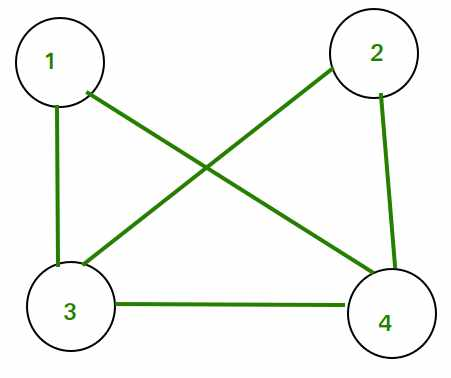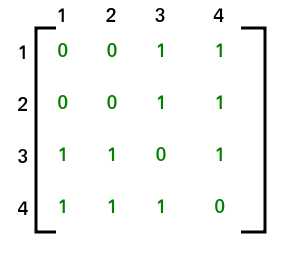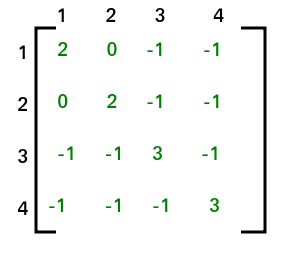# Total number of Spanning Trees in a Graph

• Difficulty Level : Hard
• Last Updated : 06 Jul, 2022

If a graph is a complete graph with n vertices, then total number of spanning trees is n(n-2) where n is the number of nodes in the graph. In complete graph, the task is equal to counting different labeled trees with n nodes for which have Cayley’s formula.

What if graph is not complete?• STEP 1: Create Adjacency Matrix for the given graph.
• STEP 2: Replace all the diagonal elements with the degree of nodes. For eg. element at (1,1) position of adjacency matrix will be replaced by the degree of node 1, element at (2,2) position of adjacency matrix will be replaced by the degree of node 2, and so on.
• STEP 3: Replace all non-diagonal 1’s with -1.
• STEP 4: Calculate co-factor for any element.
• STEP 5: The cofactor that you get is the total number of spanning tree for that graph.

Consider the following graph:Adjacency Matrix for the above graph will be as follows:After applying STEP 2 and STEP 3, adjacency matrix will look likeThe co-factor for (1, 1) is 8. Hence total no. of spanning tree that can be formed is 8.

NOTE: Co-factor for all the elements will be same. Hence we can compute co-factor for any element of the matrix. This method is also known as Kirchhoff’s Theorem. It can be applied to complete graphs also.

This article is contributed by Kapil Khandelwal. If you like GeeksforGeeks and would like to contribute, you can also write an article and mail your article to review-team@geeksforgeeks.org. See your article appearing on the GeeksforGeeks main page and help other Geeks.

My Personal Notes arrow_drop_up